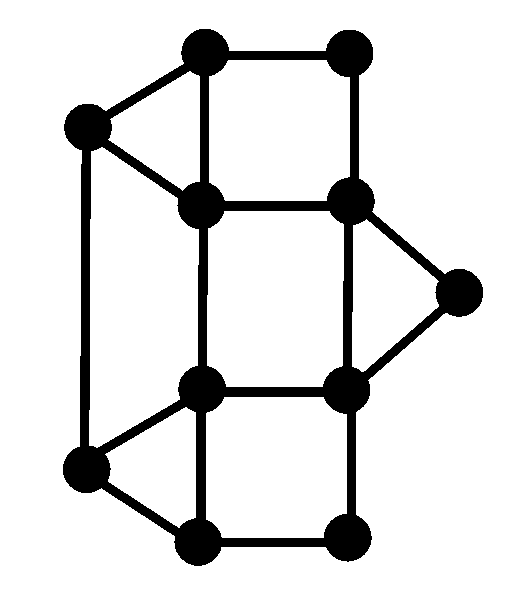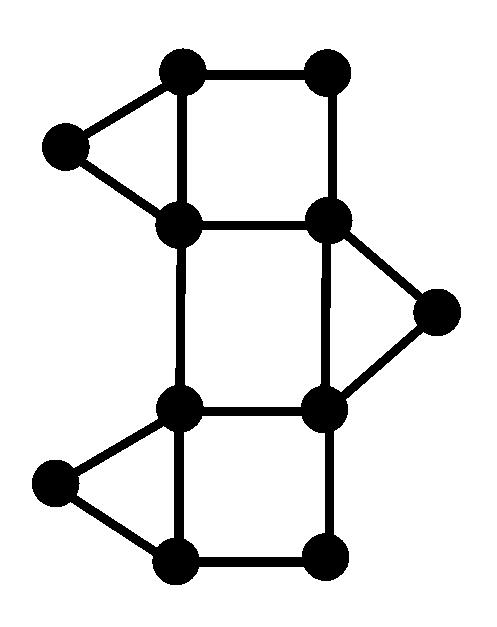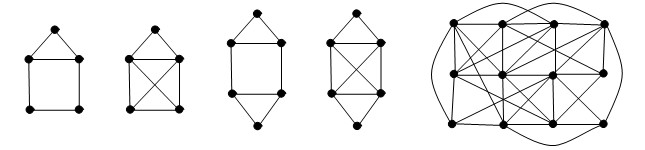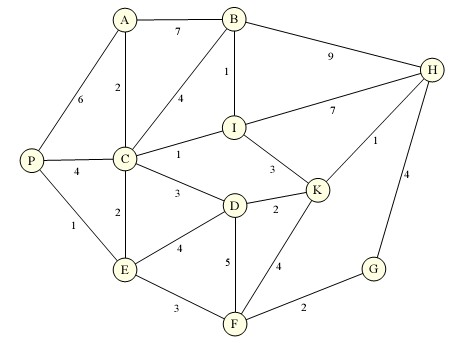# Graphs: Eulerian ToursThe streets of a small village are modeled as a graph, like the one shown to the right. The post car has to drive through all streets every day. Is it possible to find a route that covers every street (edge) exactly once? This is called an Eulerian Tour. If the tour starts where it started, it is called closed, otherwise open. A graph is called Eulerian if it allows a closed or open Eulerian tour.

See the famous "house of the Nicolaus" as an example.We face two problems:

1. How can we recognize Eulerian graphs?
2. Even if we know that a given graph is Eulerian, i.e. allows an Eulerian tour, how do we find this tour?

## Eulerian Graphs

The first problem has to do with the degrees of the vertices. Assume we have an Eulerian tour in a graph. Consider any vertex x that is not the start vertex. At the beginning, all d(x) edges incident with x are untraveled. After having visited x the first time, and having left, two of these edges incident with x have been traveled so far, thus there remain d(x)-2 untraveled edges incident with x. Note that we have to return to x as long as there are untraveled edges incident with x, since we are assuming we are travelling an Eulerian tour. This continues, until eventually we have 1 or 2 untraveled edges incident with x, depending on whether the degree d(x) of x is odd or even. In the first case, as soon as we return to x, we have to stop there. Thus in this case x must be the end vertex of the tour. Thus, if an Eulerian tour is possible, all vertices of odd degree must either be the start or the end vertex of that tour. Therefore, at most 2 of such vertices of degree 2 are possible.

Theorem [Euler 1736]: A graph allows a Eulerian tour if it is connected and has at most two vertices of odd degree. If there are such vertices of odd degree, the Eulerian tour has to start in one of them and end in the other.

For example, look at the following graphs and test which of them has a Eulerian tour:## Finding the Eulerian Tour in Eulerian Graphs

Actually we didn't prove the whole theorem yet, just that the condition is necessary. We will show sufficiency of the condition algorithmically, providing an algorithm (called "EULERTOUR") that finds an Eulerian tour if the two conditions are satisfied. This addresses also the second problem mentioned above. We have to describe an algorithm simple enough that every five-year old, when sticking to it, would succeed in finding such a Euler tour. Let's begin with an obvious one, which however, as we will see, will not always be successful.

Algorithm JUSTGO: If there are two vertices of odd degree, start at one of them, otherwise start at any vertex. Now proceed to travel untraveled edges as long as possible.If you click on "start" here , the algorithm JUSTGO will be performed. We choose to start at one of the two odd-degree vertices diplayed in refd on the algorithm. We get stuck at the second odd-degree vertex.

To resolve this problem, and improve the tour we have found so far, we proceed as follows: Look at the graph G2 having the same vertices as the original one G, but having only those edges that do not belong to the tour chosen so far. This graph G2 is not connected, but all vertices have even degree. (Why? ...) We go to the last vertex of the tour, let's call it z, that has still edges outside the tour incident. Using JUSTGO on G2, we start at z and move. Since all vertices have even degree in G2, and passing any vertex reduces the number of still available edges at that vertex by 2, we cannot get stuck but at z. So eventually we will come back to z. Now all we need to do is to insert this detour into our initial tour, making it a little longer. As long as this longer tour doesn't still cover all edges, we continue in this way: and and and and to eventually find our Eulerian tour.

Algorithm ADDLOOP: Assume we have a connected graph with
• either no odd-degree vertex, and x=y any vertex, or
• exactly two odd-degree vertices x and y.
and assume we have a tour from x to y, using every edge at most once, and not using some edges (in other words, it is not an Eulerian tour). Then we can extend the length of this tour as follows:
1. Find some vertex z on the tour with some of its incident edges not traveled by the tour yet.
2. Use algorithm JUSTGO, starting on z and using only edges not traveled by the tour considered yet, to find a tour going back to z.
3. Insert this detour in the existing tour at z.

Therefore, for every graph with at most two verteces of odd degree, every non-Eulerian tour starting on the right vertex (on a vertex of odd degree if that exists) can be extended. Thus eventually we will have found our Eulerian tour.

Algorithm EULERTOUR:
• Find a first incomplete tour, using JUSTGO.
• As long as the tour is not Eulerian yet (doesn't visit all edges), increase the size of the tour using ADDLOOP.A special case of this problem---whether or not it would be possible to travel through Königsberg and cross each of the seven bridges exactly once--- was solved by the Swiss mathematician Leonhard Euler (1707-1783) in 1736. He not only solved the simple special case, but also provided the general theorem below and started a whole branch of mathematics, which nowadays is called Graph Theory.

Euler spent his life working in The Russian Academy of Sciences in St Petersburg (1727-1741), and at the Academy in Berlin (1741-1766), and was called back to St Petersburg by Catherine the Great in 1766.

• .............

### Exercises

1. Which one of the three graphs below has an Eulerian tour? If there is one, is it open or closed?2. Which one of the graphs below has an Eulerian tour? If there is one, describe it by giving the sequence of vertices visted.3. Every morning the lazy postman takes the bus to the post office. Starting there, he arranges his route so that he ends at home to go back to sleep as quickly as possible. Below is a map of the streets along which he must deliver mail, giving the number of minutes required to walk each street, whether delivering or not. P denotes the post office and H denotes home. SourceWhich edges have to be traversed more than once in an optimal route (i.e. a shortes tour from P to H containing all edges)?
4. Let a graph have all three digit numbers from 0 to 999 (including those with leading zeros, like 000, 001, 002, ... 010, 011, ... as vertices. Two such vertices are adjacent if they share at least one digit at the same position (example 132 and 038 are adjacent). What is the degree of a vertex in the graph? Is the graph Eulerian?
5. Let a graph have all three digit numbers from 0 to 999 (including those with leading zeros, like 000, 001, 002, ... 010, 011, ... as vertices. Two such vertices are adjacent if they share two digits at the same position (example 138 and 038 are adjacent). What is the degree of a vertex in the graph? Is the graph Eulerian?